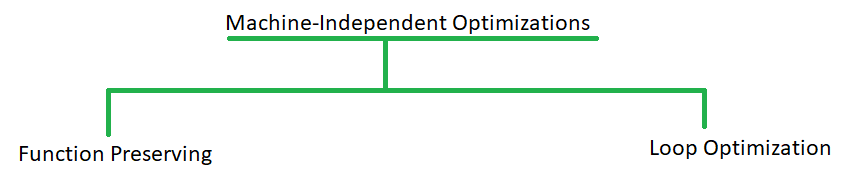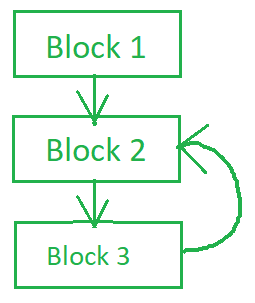# Machine Independent Code optimization in Compiler Design

• Difficulty Level : Hard
• Last Updated : 16 Sep, 2021

Machine Independent code optimization tries to make the intermediate code more efficient by transforming a section of code that doesn’t involve hardware components like CPU registers or any absolute memory location. Generally, it optimizes code by eliminating redundancies, reducing the number of lines in code, eliminating useless code or reducing the frequency of repeated code. Thus can be used on any processor irrespective of machine specifications.

Machine independent code optimization can be achieved using the following methods:Function Preserving  Optimization :

Function Preserving optimizations deals with the code in a given function in an attempt of reducing the computational time. It can be achieved by following methods:

1. Common Subexpression elimination
2. Folding
4. Copy Propagation

1. Common Subexpression elimination :

A common subexpression is the one which was computed and doesn’t change after it last computation, but is often repeated in the program. The compiler evaluates its value even if it does not change. Such evaluations result in wastage of resources and time. Thus it better be eliminated. Consider an example:

```//Code snippet in three address code format
t1=x*z;
t2=a+b;
t3=p%t2;
t4=x*z;     //t4 and t1 is same expression
//but evaluated twice by compiler.
t5=a-z;

// after Optimization
t1=x*z;
t2=a+b;
t3=p%t2;
t5=a-z;```

It is troublesome if a common subexpression is often repeated in a program. Thus it needs to be eliminated.

2.  Constant Folding :

Constant Folding is a technique where the expression which is computed at compile time is replaced by its value. Generally, such expressions are evaluated at runtime, but if we replace them with their values they need not be evaluated at runtime, saving time.

```//Code segment
int x= 5+7+c;

//Folding applied
int x=12+c;```

Folding can be applied on boolean, integers as well as on floating point numbers but one should be careful with floating point numbers. Constant folding is often interleaved with constant propagation.

Constant Propagation :

If any variable is assigned a constant value and used in further computations, constant propagation suggests using the constant value directly for further computations. Consider the below example

```// Code segment
int a = 5;
int c = b * 2;
int z = a;

//Applying constant propagation once
int c = 5 * 2;
int z = a;

//Applying constant propagation second time
int c = 10;
int z = a;

//Applying constant propagation last time
int z = a;```

Dead code is a program snippet that is never executed or never reached in a program. It is a code that can be efficiently removed from the program without affecting any other part of the program. In case, a value is obtained and never used in the future, it is also regarded as dead code. Consider the below dead code:

```//Code
int x= a+23;  //the variable x is never used
//in the program. Thus it is a dead code.
z=a+y;
printf("%d,%d".z,y);

//After Optimization
z=a+y;
printf("%d,%d".z,y);```

Another example of dead code is assign a value to a variable and changing that value just before using it. The previous value assignment statement is dead code. Such dead code needs to be deleted in order to achieve optimization.

4. Copy Propagation :

Copy Propagation suggests to use one variable instead of other, in cases where assignments of the form x=y are used. These assignments are copy statements. We can efficiently use y at all required place instead of assign it to x. In short, elimination of copies in the code is Copy Propagation.

```//Code segment
----;
a=b;
z=a+x;
x=z-b;
----;

//After Optimization
----;
z=b+x;
x=z-b;
----;```

Another kind of optimization, loop optimization deals with reducing the time a program spends inside a loop.

Loop Optimizations :

The program spends most of its time inside the loops. Thus the loops determine the time complexity of the program. So, in order to get an optimal and efficient code, loop optimization is required. In order to apply loop optimization, we first need to detect the loop using control flow analysis with the help of program flow graph. A cycle in a program flow graph will indicate presence of a loop. Note that, the code from Intermediate code Generation phase which is in three-address format is given as input to the optimization phase. It is difficult to identify loops in such a format, thus a program flow graph is required.

The program flow graph consists of basic blocks, which is nothing but the code divided into parts or blocks and show the execution flow of the code,Sample Program flow graph

The cycle in the above graph shows a presence of loop from block 2 to block3.

Once the loops are detected following Loop Optimization techniques can be applied :

1. Frequency Reduction
2. Algebraic expression simplification
3. Strength Reduction
4. Redundancy Elimination

1. Frequency Reduction :

It applies to the concept that a loop runs for least possible lines of code. It can be achieved by following methods:

a. Code Motion :

Many times, in a loop, statements that remain unchanged for every iteration are included in the loop. Such statements are loop invariants and only result in the program spending more time inside the loop. Code motion simply moves loop invariant code outside the loop, reducing the time spent inside the loop. To understand this consider the example below.

```//Before code motion

p=100
for(i=0;i<p;i++)
{
a=b+40;         //loop invariant code
if(p/a==0)
printf("%d",p);
}

// After code motion

p=100
a=b+40;
for(i=0;i<p;i++)
{
if(p/a==0)
printf("%d",p);
}```

In the example, before optimizing, the loop invariant code was evaluated for every iteration of the loop. Once code motion is applied, the frequency of evaluating loop invariant code also decreases. Thus it is also called as Frequency Reduction. The following is also an example for code motion.

```//Before code motion
----;
while((x+y)>n)
{
----;
}
----;

// After code motion
----;
int t=x+y;
while(t>n)
{
----;
}
----;```

b. Loop Unrolling :

If a loop runs doing the same operation for every iteration, we can perform that same operation inside the loop more than once. This is called loop unrolling. Such unrolled loop will perform the evaluation more than once in a single loop iteration.

```//Before Loop unrolling

while(i<50)       //while loop initialize all array elements to 0;
//one element each iteration. Thus the loop runs 50 times.
{
x[i]=0;
i++;
}

//After loop unrolling

while(i<50)       //After unrolling, each iteration
//initializes 5 elements to 0;
//Thus this loop runs only 5 times.
{
x[i]=0;
i++;
x[i]=0;
i++;
x[i]=0;
i++;
x[i]=0;
i++;
x[i]=0;
i++;
}```

As in above example , an unrolled loop is more efficient than the previous loop.

c. Loop Jamming :

Combining the loops that carry out the same operations is called as loop jamming.

```//Before loop jamming
----;
for(i=0;i<5;i++)      //Setting all elements of 2D array to 0.
{
for(j=0;j<5;j++)
{
x[i][j]=0;

}
}
for(i=0;i<5;i++)     //Setting diagonal elements to 1.
{
x[i][i]=0;

}
----;

//After loop jamming
----;
for(i=0;i<5;i++)      //Setting all elements of 2D array to 0
//and diagonal elements to 1.
{
for(j=0;j<5;j++)
{
x[i][j]=0;
x[i][i]=1;
}
}
----;```

Thus, instead of executing same loops twice, that operation can be done by executing the loop only once.

2. Algebraic expression simplification :

A program might contain some trivial algebraic expressions that do not result in any useful computation or change of value. Such lines of code can be eliminated so the compiler doesn’t waste time evaluating it. For example,

```A=A+0;
x=x*1;```

These statements do not result in any useful computations. Such code may seem harmless, but when used inside any loops they keep on being evaluated by compiler. Thus it is best to eliminate them.

3. Strength Reduction :

It suggests replacing a costly operation like multiplication with a cheaper one.

```Example:
a*4

after reduction
a<<2```

It is an important optimization for programs where array accesses occur within loops and should be used with integer operands only.

4. Redundancy Elimination :

It may happen, that a specific expression is repeated in a code many times. This expression is redundant to code because we may evaluate it once and substitute its next occurrence with its evaluated value. This substitution is nothing but redundancy elimination. A simple example is given below

```//Code:
----;
int x=a+b;
----;
int z=a+b+40;
----;
return (a+b)*5;

//After optimization
----;
int x=a+b;
----;
int z=x+40;
----;
return x*5```

Redundancy elimination avoids evaluating the same expressions multiple times resulting in faster execution.

My Personal Notes arrow_drop_up